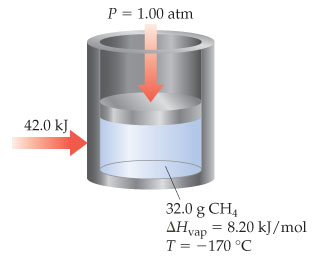# Problem: If 42.0 kJ of heat is added to a 32.0-g sample of liquid methane under 1 atm of pressure at a temperature of -170oC, what is the final state of the methane once the system equilibrates? Assume no heat is lost to the surroundings. The normal boiling point of methane is -161.5 oC. The specific heats of liquid and gaseous methane are 3.48 and 2.22 J / g, respectively.

81% (52 ratings)
###### Problem Details

If 42.0 kJ of heat is added to a 32.0-g sample of liquid methane under 1 atm of pressure at a temperature of -170oC, what is the final state of the methane once the system equilibrates? Assume no heat is lost to the surroundings. The normal boiling point of methane is -161.5 oC. The specific heats of liquid and gaseous methane are 3.48 and 2.22 J / g, respectively.